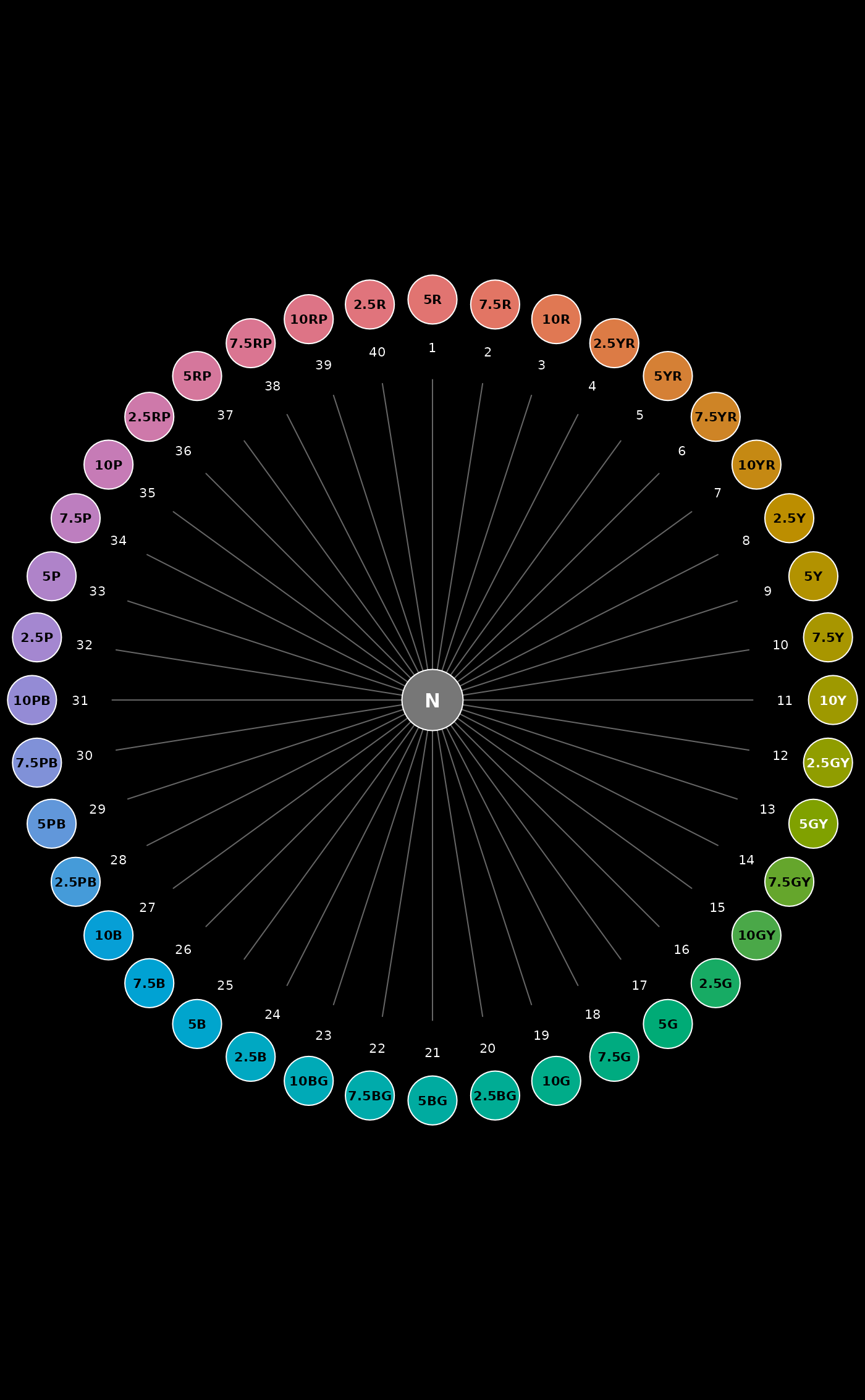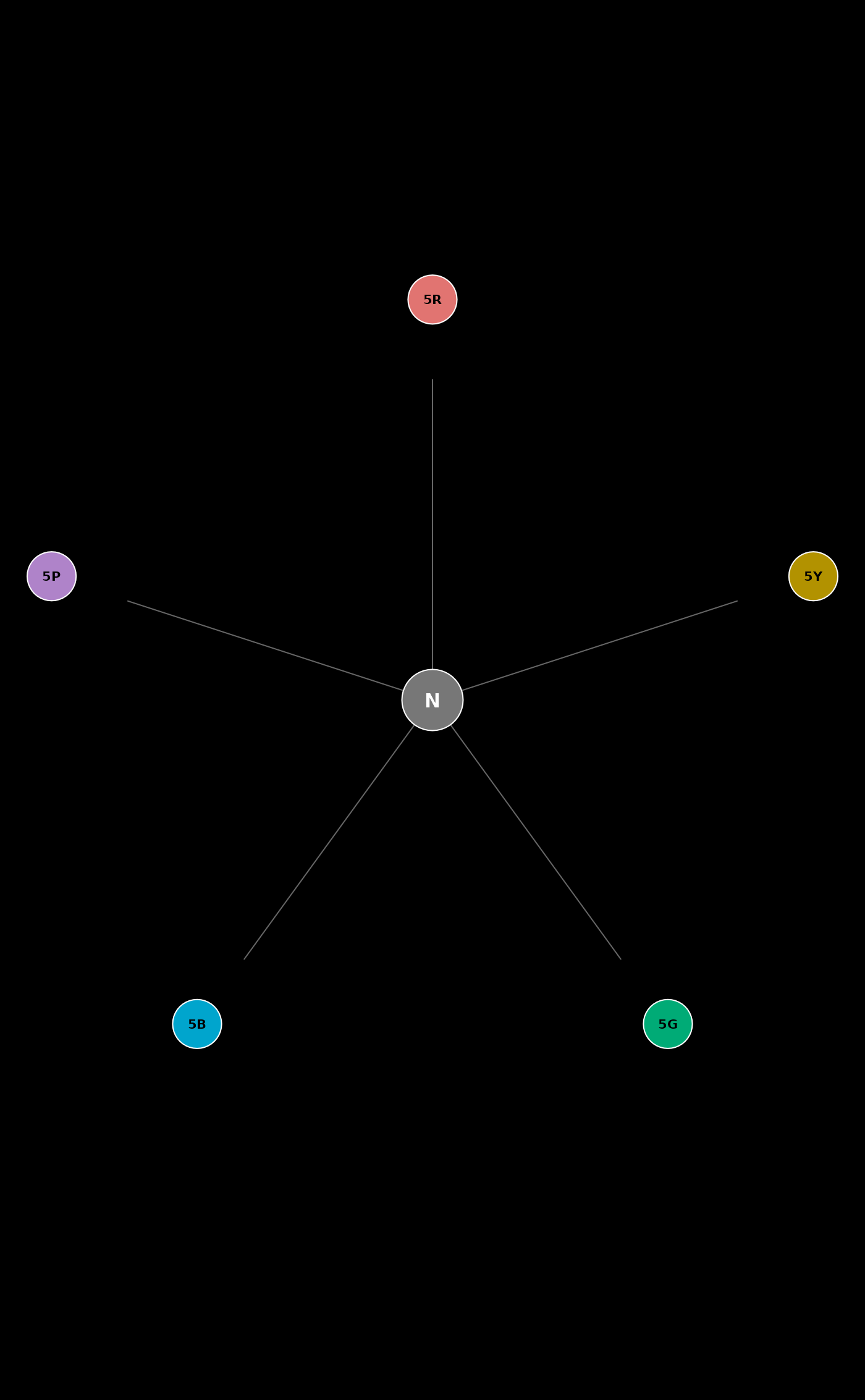Munsell hues are arranged on the unit circle with "neutral" at the center.

huePositionCircle(
hues = huePosition(returnHues = TRUE),
value = 6,
chroma = 10,
chip.cex = 5.5,
label.cex = 0.66,
seg.col = "grey",
plot = TRUE,
simulateCVD = NULL,
CVDseverity = 1
)

## Arguments

hues

vector of Munsell hues, commonly derived from huePosition()

value

single integer, Munsell value used to create an actual color

chroma

single integer, Munsell chroma used to create an actual color

chip.cex

numeric, scaling for color chips

label.cex

numeric, scaling labels

numeric, scaling for line segment cues

seg.col

single color, color used for line segment cues

plot

logical, generate output on the current graphics device

simulateCVD

simulate color vision deficiencies with the colorspace package, should be the character representation of a function name, one of: 'deutan', 'protan', or 'tritan'.

CVDseverity

numeric value between 0 (none) and 1 (total), describing the severity of the color vision deficiency

## Value

an invisible data.frame of data used to create the figure

## Note

The best results are obtained when setting margins to zero, and inverting foreground / background colors. For example: par(mar = c(0, 0, 0, 0), fg = 'white', bg = 'black').

## Examples


# better graphics defaults
op <- par(mar = c(0, 0, 0, 0), fg = 'white', bg = 'black')

# full set of hues, as generated by huePosition(returnHues = TRUE)
huePositionCircle()# subset
huePositionCircle(hues = c('5R', '5Y', '5G', '5B', '5P'))# reset graphics state
par(op)